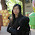## Wednesday, April 24, 2013

### Help: I caught a bug

I think I must be turning too much into a statistician and econometrician lately, I must have caught a virus or something. Maybe it started already a while ago

The theme of the scipy conference this year is "Machine Learning & Tools for Reproducible Science". However, I'm not doing any sexy twitter analysis, I just spent some days coding tests for proportion, boring stuff like pairwise comparisons of proportions.

Anyway, I decided to submit a tutorial proposal for econometrics with statsmodels to the scipy conference, see (lightly edited) proposal below. Since my proposal didn't get accepted, my first response was: Wrong topic, Too much statistic, We just want numbers, not check whether the model is correct, and find out how to fix it.

That leaves me with more time to go back to figuring out which other basic statistical tests are still missing in Python.

#### Description

This tutorial will give an overview of statsmodels and an introduction to the usage of it for statistical analysis. Special emphasis will be given to the choice of models and specification and diagnostic issues. After an introduction to statsmodels, we will look at cases where the basic linear model is not appropriate. We will use statistical tests and graphical tools to identify possible specification problems, and show which alternative models are available and how those can be used.

Throughout the tutorial I will emphasize the statistical background and assumptions that each model has, so we get estimators with good properties and valid inference.

The tutorial assumes that users have some basic or intermediate knowledge of working with numpy, and some basic knowledge of statistics. Statistical concepts will be introduced and used on a relatively basic level. Each section will include examples and exercises.

The tutorial should enable participants to use statsmodels for their statistical analysis, and make them aware of the capabilities of statsmodels in cases where a model might not be appropriate or correctly specified.

#### Part 1: Introduction to Statsmodels

After a short broad overview, we will introduce the basic usage of statsmodels using two of the most commonly used models, OLS and Logit. We will show and use the integration of statsmodels with pandas for data handling and with patsy for the formula interface.

We will also include a brief introduction to statistical tests and power analysis that are in statsmodels as a complement to scipy.stats.

#### Part 2: Do we have the right model?

In this part we will use statsmodels to check or test whether our model is appropriate for our dataset, and for the case when it is not, we will consider ways to adjust our analysis or consider alternative models that are more appropriate. For each case, we will use the graphical tools and statistical tests that statsmodels provides to verify our model specification, and to use an alternative model that is more appropriate. We will go into details for some of the following cases, and only touch others.

Introduction to model assumptions, and properties of estimators and inference

Outliers: detection and robust estimation (RLM)

Heteroscedasticity (unknown non-constant variance): detection, robust standard errors, estimating variance

Autocorrelation (serially correlated errors): detection, robust standard errors, and alternative estimators GLSAR, ARMAX

Normality: qqplots, goodness-of-fit tests, Is it important?

Nonlinearity: detection, nonlinear models

Missing exogeneity: explanatory variables are not independent of process that generated the dependent variable. Instrumental Variable estimation

Multicollinearity and large number of explanatory variables: detection, L1 penalized discrete models

Part 3: Outlook

brief show case: quantile regression, non-parametric estimation The future of statistics in python.

1.I am very interested in the content of your proposed tutorial. It is very useful for research students like me that don't have a good statistic background. Although the tutorial was not accepted by scipy, it is still awesome if it could be published as a series of blog posts!

2.Hi Andy,

Thank you for the interest in this. I'm still planning to write these, but, now, with less time pressure.

I still have two or three additional posts on hypothesis testing in my plans, before going back to those topics.

3.Maybe you could submit the tutorial to another conference? It's an interesting topic. pycon.ca is later this year, and I think they are about to post their CFP dates.